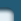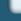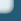Lin.-Quad. Control

Web design by Alexei Kuntsevich## Selected Publications


F. Kappel and D. Salamon. Spline approximation for retarded systems and the Riccati equation. SIAM J. Control and Optimization, 25:1082–1117, 1987.


F. Kappel and D. Salamon. Approximation of the algebraic Riccati operator equation. In Proc. 27th IEEE Conference on Decision and Control, December 7–9, 1988, Austin, Texas, pages 2001–2002, 1988.


F. Kappel and D. Salamon. An approximation theorem for the algebraic Riccati equation. SIAM J. Control and Optimization, 28:1136–1147, 1990.

For an infinite dimensional linear quadratic control problem in Hilbert space, approximation of the solution of the algebraic Riccati operator equation in the strong operator topology is considered under conditions weaker than uniform exponential stability of the approximating systems. As an application strong convergence of the approximating Riccati operators in case of a previously developed spline approximation scheme for delay systems is established. Finally we investigate convergence of the transfer-functions of the approximating systems.


F. Kappel. Approximation of LQR-problems for delay systems: a survey. In K. Bowers and J. Lund, editors, Computation and Control II, pages 187–224, Boston, 1991. Birkhäuser.